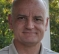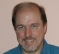## Estimating SNR without windowingStarted by 6 years ago10 replieslatest reply 6 years ago227 views

I've come across several wonderful algorithms for estimating the parameters of a complex tone in noise (ex. Macleod, Jacobsen, Flat Top window, etc), that I've simulated in software. However, I also need to estimate the SNR. I came up with a simple formula based on my intuition that the correlation of simulated gaussian noise with a tone is zero (thus I could just subtract the tone power from the total power to get the noise power). The formula I tried was thus $$SNR = \frac{A^2}{\sum |X|^2/N^2 -A^2}$$

where $$A$$ is the estimated time-domain amplitude of the tone and $$X$$ is the DFT of the tone + noise.

This seems to work well for low SNR, but starts doing poorly at SNR's greater than 25 dB or so. In fact, the denominator will sometimes come up negative, in which case the result is NaN. I suspect the issue is due to quantization/floating point arithmetic error.

Alternatively, I also tried windowing my time-domain data with a Hanning window and estimating my noise power by measuring the noise floor with a mean in the frequency dB domain. This approach works far better. However, the only tone parameter estimation methods I've seen that rely on windowed data are Grandke's for frequency and quadratic interpolation for amplitude. These are fine, but they do not perform as well in simulation as the others.

So my question is if there are any better ways of calculating SNR from rectangular windowed data, or alternatively if there are parameter estimators that work as well as estimators that assume rectangular windowing. It would be kind of disappointing if I end up not being able to take advantage of all these interesting estimators!

[ - ]These are my articles on frequency estimation for a pure complex tone usng a DFT:

A Two Bin Exact Frequency Formula for a Pure Complex Tone in a DFT
https://www.dsprelated.com/showarticle/1039.php

Three Bin Exact Frequency Formulas for a Pure Complex Tone in a DFT
https://www.dsprelated.com/showarticle/1043.php

My (admittedly limited) testing has shown them to be superior to the ones you mentioned.

To find the SNR, I would take fundamental definition approach.  First find the amplitude and phase of your signal.  This is equivalent to finding a complex coefficient for a basis function made using your estimated frequency.  You can then construct an estimated signal using the parameters you have found.  Your best assumption is that this is the true underlying signal.  It will not be, of course, but it should be as close as you can find.  Then subtract your constructed signal from your sampled signal to find the noise signal.  The SNR should be the ratio of the power of the constructed signal to the power of the noise signal.

Hope this helps,
Ced

[ - ]I have used you fundamental approach several times.

I have even used it for data communications, where I synthesized a transmit signal, and manually tinkered with the parameters while watching the level of the signal after the subtraction to minimize it.  Results in estimation of all sorts of things at the same time.

Timing frequency offset, timing phase recovery, carrier frequency offset, carrier phase, pulse shaping filter, equalization, etc. etc.

Works for me.

David

[ - ]It should be easy to compute the total power in the input, then estimate the power in the tone from the amplitude estimate you determine, the noise power is then just the difference.  It will be an estimate, but a blind estimate of SNR is always difficult to determine with high precision.

Are you most interested in estimating power, phase, or frequency of the input?   Or everything?

There's been a fair amount of newer work for estimators, and depending on what you really want or what your tolerance is for complexity may narrow down the selections.  Cagatay Candan's work is popular and provides options on increasing complexity for better performance.

[ - ]Candan's 2013 formula is compared in my three bin article.  One of my new formulas is equivalent and the others are better.

In the approach I gave in my previous answer, the constructed signal will be orthogonal to the noise signal.  Therefore

Power of Sampled = Power of Constructed + Power of Noise

You can find an amplitude estimate from the DFT by constructing a basis vector in DFT space using the formulas in my article:

DFT Bin Value Formulas for Pure Complex Tones
https://www.dsprelated.com/showarticle/1038.php

The approach is a one dimensional version of the technique I demonstrate for real tones in my article:

Phase and Amplitude Calculation for a Pure Real Tone in a DFT: Method 1
https://www.dsprelated.com/showarticle/787.php

Regards,
Ced
[ - ]But that's what he's doing with his formula, basically.

[ - ]I would replace A by A/2 in your formula and sum over positive frequencies only (which maybe you're already doing).  The FFT bin magnitude is N*A/2 not N*A in the DFT of an amplitude A sinusoid tuned to a bin frequency.  Even if you are summing the DFT squared-magnitude over all N bins, (A/2)^2 + (A/2)^2 = A^2/2 not A^2.  You can still get NaN if the noise happens to push the peak(s) down, so more robust methods are better to use.

I like to remove the sinusoids from the spectrum to leave what is called a "residual spectrum" (search for "sinusoidal modeling" or "sines plus noise spectral modeling"), then estimate the envelope of the remaining noise floor in a way that fills in any "hole" left by removing the sinusoid.  Finally, use the mean height of that noise-floor estimate (or the area under it) to determine my noise variance estimate.

[ - ]The OP has a single complex tone signal.  Your answer appears to be for a real tone.

Ced

[ - ]I found an easy solution for SNR for my particular problem.  In my case the noise tends to be broadband, and could have a pink noise spectrum.  My solution is to use two bandpass filters.  One is narrow band to pass the signal.  The other is a rather broad band to capture both signal and noise.  The ratio of output powers of these two filters is just Signal to Signal-Plus-Noise.  SNR is easily computed from this ratio.

[ - ]Looking at your equation again, it seems you are doing the same calculation we recommended.  Therefore, you're problem is probably how you are calculating "A".

Could you post your method for finding "A"?

Ced

[ - ]Your problem is that the formula is subject to errors in calculating $$A$$, and if you overestimate it, then you can get your negative number in the denominator.
A better way to do it would be to calculate the strength of the signal with the tone subtracted out.  I would either calculate the average power in all of the bins of $$X$$ except those right around the tone frequency, or I would calculate $$y_k = x_k - A e^{\theta k}$$, where $$\theta$$ is the normalized frequency in radians per sample, and use $$\frac{\sum \left | Y \right | ^2}{N^2}$$ as the denominator.